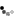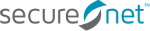Loading... Please wait...

# Safe Balancing

## Safe Balancing

INTRODUCTION - To correctly and safely load vehicles on your lift, it is necessary to determine the weight and Center of Balance (CB) for each vehicle being lifted.

The acronyms and terms used in Figure 1 and elsewhere to compute CB are as follows:

• FOH - (front overhang) distance in inches from front bumper to center of front axle.
• WB - (wheel base) distance from center of front axle to center of rear axle or center of tandem axles.
• FAW - front axle weight in pounds.
• RAW - rear axle weight in pounds.
• GW - gross weight in pounds.
• RDL - (reference datum line) predetermined point from which all measurements are taken.
• MOMENT - the product obtained by multiplying the weight by the distance from the RDL.
• W1 - front axle weight
• W2 - rear axle weight
• D1 - distance from RDL to front axle
• D2 - distance from RDL to rear axle

Fig. 1Figure 1 illustrates the vehicle measurement points that must be used to calculate the CB of a vehicle.

EXAMPLE / SINGLE AXLE VEHICLE

STEP 1.
Determine front and rear axle weights and gross weight.• W1 = front axle weight (3,500 lbs.)
• W2 = rear axle weight (5,500 lbs.)
• Gross Weight = 3,500 + 5,500 (9,000 lbs.)VEHICLE MEASUREMENTS - If axle weights are not accurately determined, and/or weight scales are not available, DO NOT lift the vehicle until axle weights are confirmed. If no data plate is found, contact the vehicle manufacturer directly or refer to the vehicle operator’s manual.

STEP 2. Determine distance from the front and rear axles to the RDL.• D1 = distance from RDL to front axle (40”)
• D2 = distance from RDL to rear axle (195”)

STEP 3. Enter the information obtained in steps 1 and 2 into the formula to determine the moment.

(W1 x D1) + (W2 x D2) = TOTAL MOMENT
(3,500 x 40) + (5,500 x 195) = TOTAL MOMENT
(140,000) + (1,072,500) = 1,212,500

STEP 4. Divide the total moment by the gross weight.

MOMENT ÷ GROSS WEIGHT = CB

1,212,500 ÷ 9,000 = 134.72"This final result (134.72”) is the CB of the vehicle measured from the reference data line (RDL).

## EXAMPLE / TANDEM AXLE TRAILER

STEP 1. Determine front and rear axle weights and gross weight.• W1 = front axle weight (5,800 lbs.)
• RAW 1 + RAW 2 = W2 rear axle weight (9,500 lbs.)
• Gross Weight = 5,800 + 9,500 (15,300 lbs.)

STEP 2. Determine distance from the front and rear axles to the RDL.• D1 = distance from RDL to front axle (52”)
• D2 = distance from RDL to CENTER of rear axles (222”)

STEP 3. Enter the information obtained in steps 1 and 2 into the formula to determine the moment.

(W1 x D1) + (W2 x D2) = TOTAL MOMENT
(5,800 x 52) + (9,500 x 222) = TOTAL MOMENT
(301,000) + (2,109,500) = 2,410,000,

STEP 4. Divide the total moment by the gross weight.

MOMENT ÷ GROSS WEIGHT = CB

2,410,000 ÷ 15,300 = 157.52"This final result (157.52”) is the CB of the vehicle measured from the reference data line (RDL).

## Popular Brands